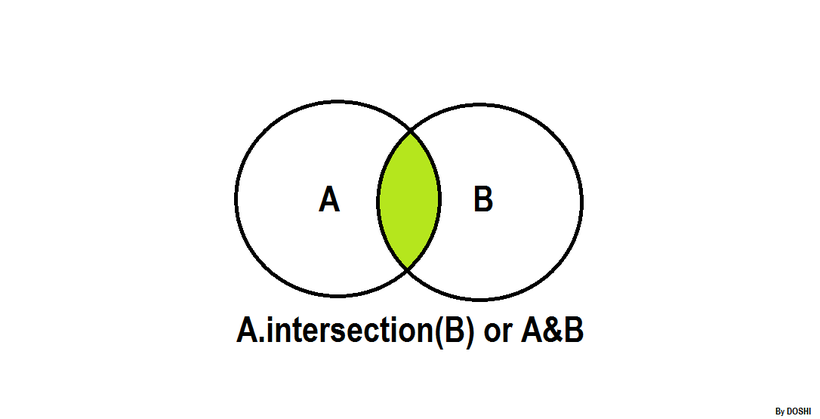## Algorithm

Problem Name: Python - Set .intersection() Operation

In this HackerRank Functions in PYTHON problem solution,.intersection()

The .intersection() operator returns the intersection of a set and the set of elements in an iterable.
Sometimes, the & operator is used in place of the .intersection() operator, but it only operates on the set of elements in set.
The set is immutable to the .intersection() operation (or & operation).

``````>>> s = set("Hacker")
>>> print s.intersection("Rank")
set(['a', 'k'])

>>> print s.intersection(set(['R', 'a', 'n', 'k']))
set(['a', 'k'])

>>> print s.intersection(['R', 'a', 'n', 'k'])
set(['a', 'k'])

>>> print s.intersection(enumerate(['R', 'a', 'n', 'k']))
set([])

>>> print s.intersection({"Rank":1})
set([])

>>> s & set("Rank")
set(['a', 'k'])
``````

The students of District College have subscriptions to English and French newspapers. Some students have subscribed only to English, some have subscribed only to French, and some have subscribed to both newspapers.

You are given two sets of student roll numbers. One set has subscribed to the English newspaper, one set has subscribed to the French newspaper. Your task is to find the total number of students who have subscribed to both newspapers.

Input Format

The first line contains n, he number of students who have subscribed to the English newspaper.
The second line contains nspace separated roll numbers of those students.
The third line contains b,the number of students who have subscribed to the French newspaper.
The fourth line contains b space separated roll numbers of those students.

Constraints

0 < Total number of students in college < 1000

Output Format

Output the total number of students who have subscriptions to both English and French newspapers.

Sample Input

``````9
1 2 3 4 5 6 7 8 9
9
10 1 2 3 11 21 55 6 8
``````

Sample Output

``````5
``````

## Code Examples

### #1 Code Example with Python Programming

```Code - Python Programming```

``````
input()
s = set(input().split())
input()
print(len(s.intersection(input().split())))
``````
Copy The Code &